# Diagonal + right triangle - math problems

#### Number of problems found: 183

• Cube diagonalDetermine the length of the cube diagonal with edge 37 mm.
• Body diagonalCalculate the length of the body diagonal of the 6cm cube.
• Body diagonalCalculate the cube volume, whose body diagonal size is 75 dm. Draw a picture and highlight the body diagonal.
• Body diagonalFind the cube surface if its body diagonal has a size of 6 cm.
• Find diagonal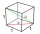Find the length of the diagonal of a cuboid with length=20m width=25m height=150m
• Median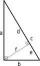In the right triangle are sides a=41 dm b=42 dm. Calculate the length of the medians tc to the hypotenuse.
• Diagonal to areaCalculate the area of a rectangle in which the length of the diagonal is 10 cm.
• Square diagonalCalculate the length of the square diagonal if the perimeter is 476 cm.
• Perimeter and diagonal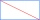The perimeter of the rectangle is 82 m, the length of its diagonal is 29 m. Find the dimensions of the rectangle.
• Square2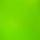Side of the square is a = 6.2 cm, how long is its diagonal?
• Space diagonalThe space diagonal of a cube is 129.91 mm. Find the lateral area, surface area and the volume of the cube.
• Diagonal BDFind the length of the diagonal BD in a rectangular trapezoid ABCD with a right angle at vertex A when/AD / = 8,1 cm and the angle DBA is 42°
• Square diagonalCalculate the length of diagonal of the square with side a = 23 cm.
• Body diagonal - cubeCalculate the surface and cube volume with body diagonal 15 cm long.
• Construct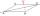Construct a rhombus ABCD, if the size of the diagonal AC is 6 cm and diagonal BD 8 cm long.
• Diagonal - simple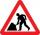Calculate the length of the diagonal of a rectangle with dimensions 5 cm and 12 cm.
• RhombusCalculate the length of the diagonal AC of the rhombus ABCD, if its perimeter is 84 dm and the other diagonal BD has length 20 dm.
• DiagonalsA diagonal of a rhombus is 20 cm long. If it's one side is 26 cm find the length of the other diagonal.
• SquareCalculate area of the square with diagonal 64 cm.
• DiagonalCalculate the diagonal length of the rectangle ABCD with sides a = 8 cm, b = 7 cm.

Do you have an interesting mathematical word problem that you can't solve it? Submit a math problem, and we can try to solve it.

We will send a solution to your e-mail address. Solved examples are also published here. Please enter the e-mail correctly and check whether you don't have a full mailbox.

Please do not submit problems from current active competitions such as Mathematical Olympiad, correspondence seminars etc...